# 17 Sample Addition And Subtraction Worksheets

Addition and subtraction form the basis of every calculation, no matter how simple or complex. Hence, the students must be encouraged to practice the addition and subtraction worksheets to improve their maths basic. The teachers are often needed to offer these worksheets to the entire class to verify the students’ strength and fundamental maths know-how.

## Sample Addition And Subtraction Worksheet Template## Three Digit Addition And Subtraction Worksheet TemplateIf your child finds adding or subtracting three digit numbers difficult, then making him practice with a Three Digit Addition and Subtraction Worksheet Template will strengthen his grip. Make him practice as many of these templates as possible to see desired results.

## Domino Addition And Subtraction Worksheet TemplateWhat is so unique about a Domino Addition and Subtraction Worksheet Template is that it comes with problems that involve addition or subtraction of dots. Small children who don’t quite understand the concept of numbers can benefit a lot from these templates.

Addition and subtraction worksheets are worksheets designed for teachers to help their students with their maths exercises. If you are in the teaching profession and are looking for worksheets for your class, you can check out addition and subtraction worksheet templates for the class. These worksheets are not only convenient, but you can also use them efficiently in your classroom. All grade students can practice their addition and subtraction exercises in the sheets. And, teachers can monitor their performance(s) in the sheets. The sheets make it easier for the teachers to check.

## Addition And Subtraction Drills Worksheet Template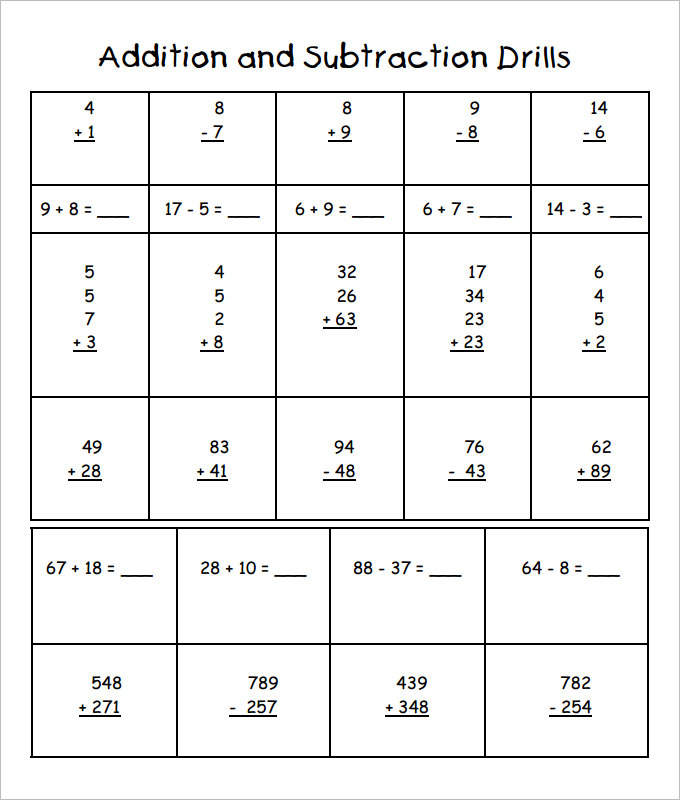## Basic Sample Addition And Subtraction Worksheet Template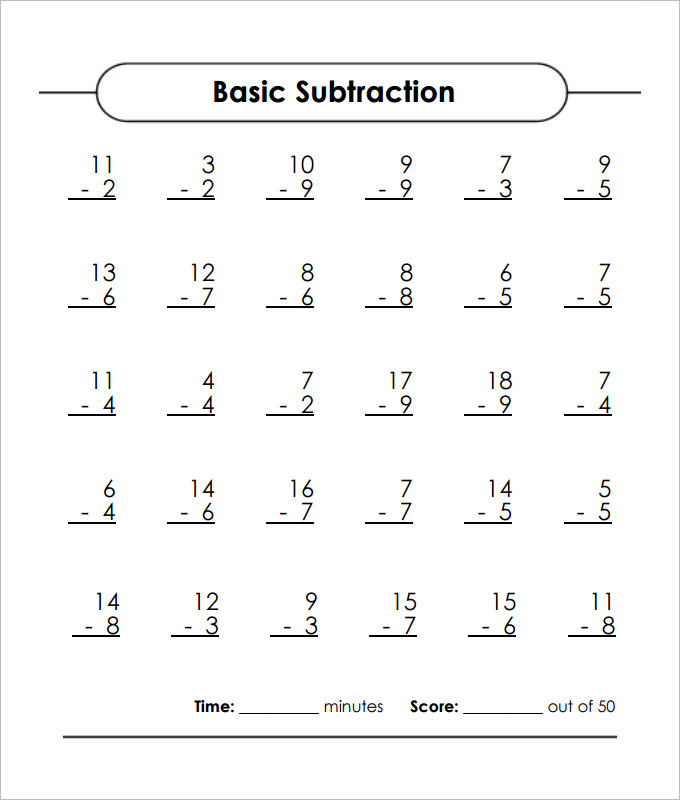## Color the Bulb Addition And Subtraction Worksheet Template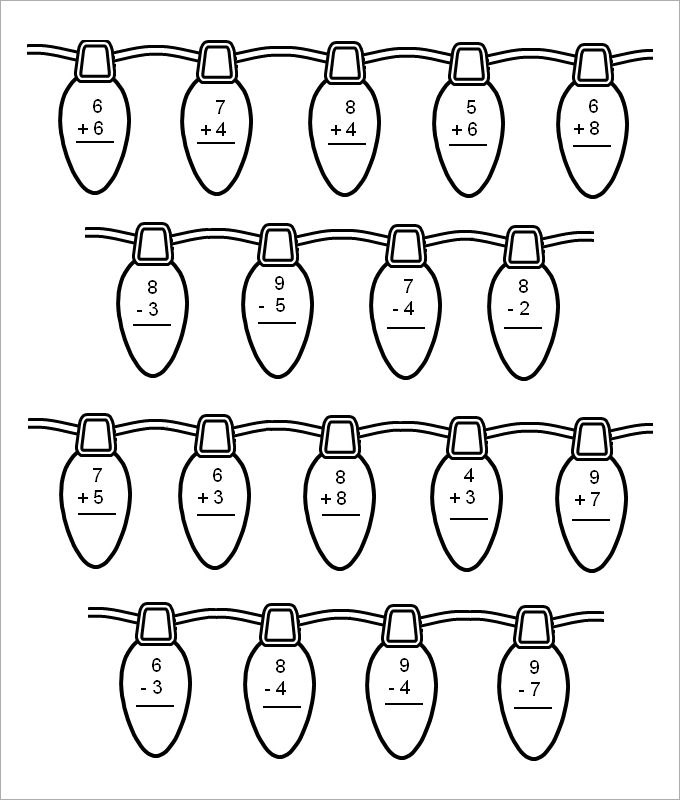This Color the Bulb Addition and Subtraction Worksheet Template, allows kids to have fun with colours apart from engaging in calculations. Just make your child practice one of these templates and you will find him enjoying his mathematics practice session more than ever.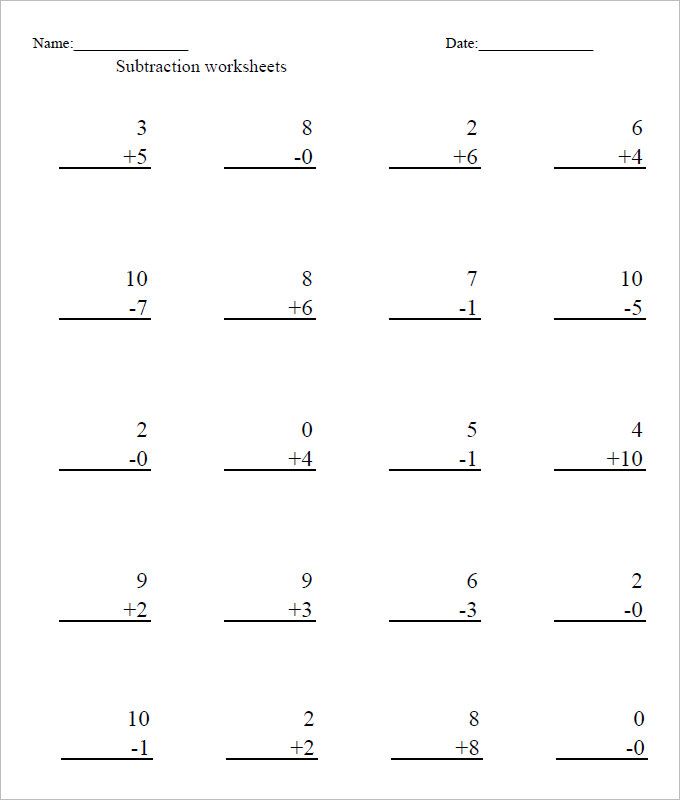## Mixed Problems Addition And Subtraction Worksheet Template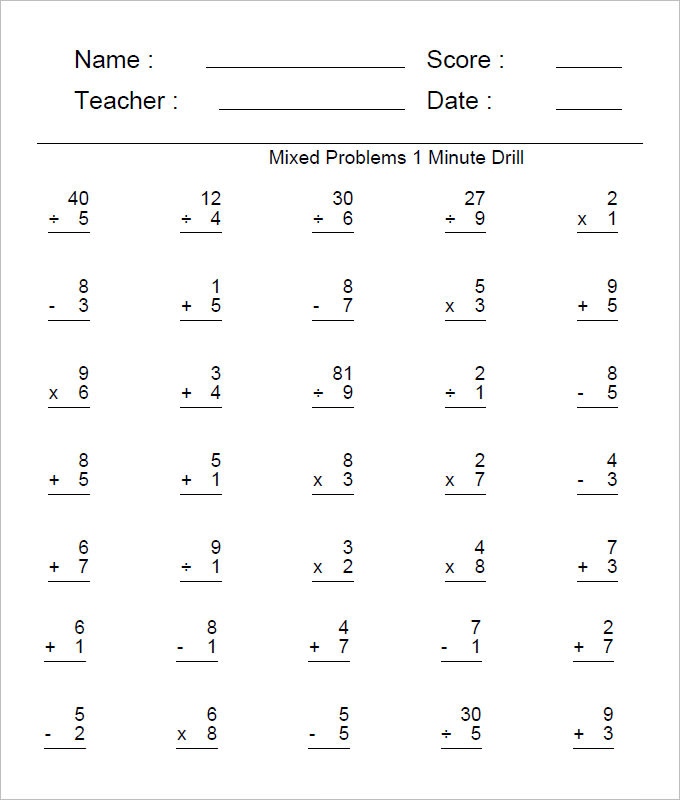## Large Addition And Subtraction Worksheet Template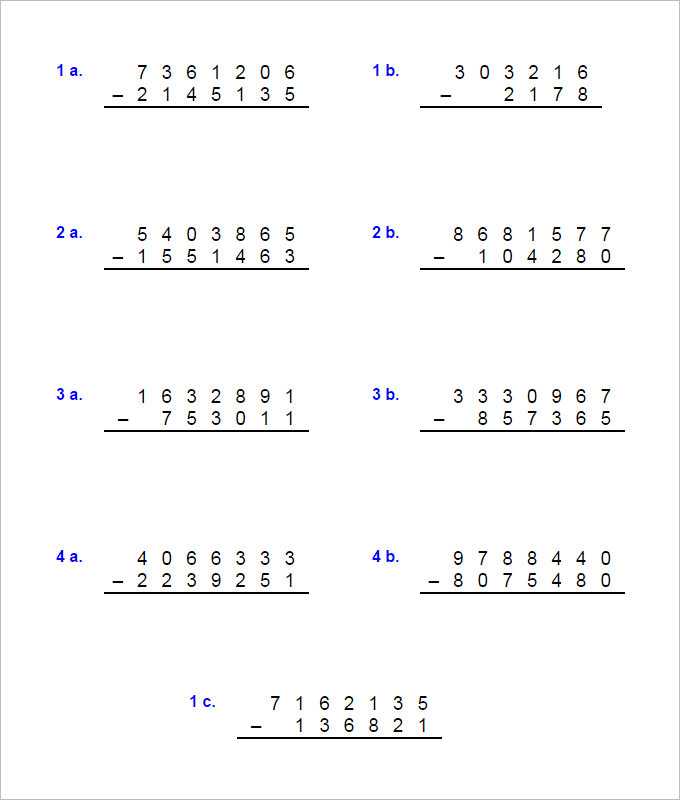This template is best suited for older kids who can solve difficult sums of addition and subtraction. This Large Addition and Subtraction Worksheet Template includes problems wherein up to seven digit numbers have been used. If your child loves challenges, then this template will highly impress him.

## Free Basic Addition And Subtraction Worksheet Template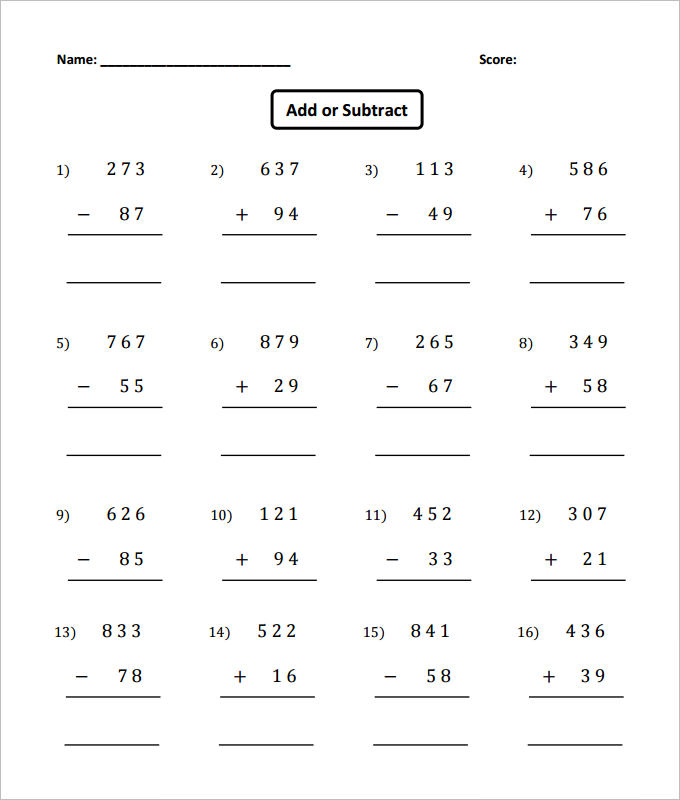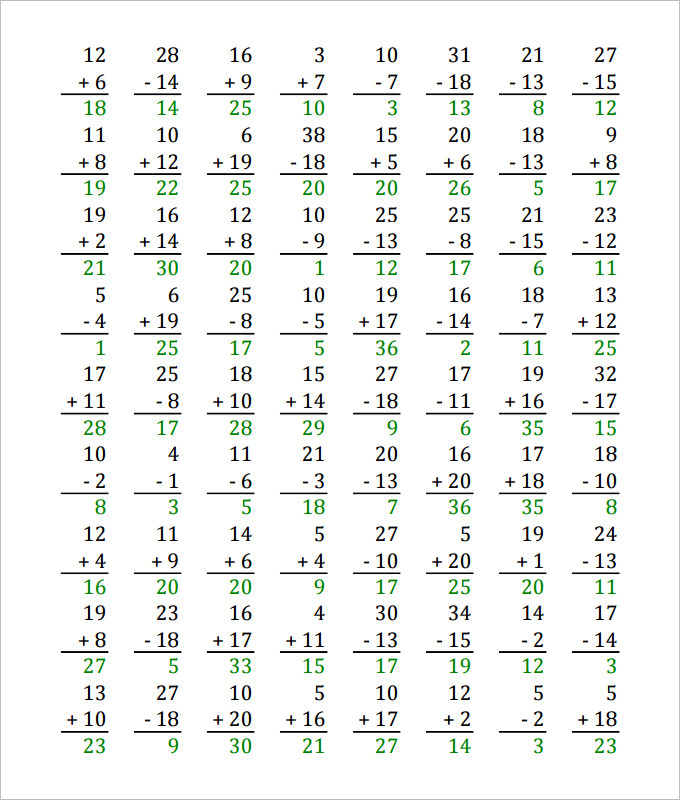## Fill the Numbers Addition And Subtraction Worksheet Template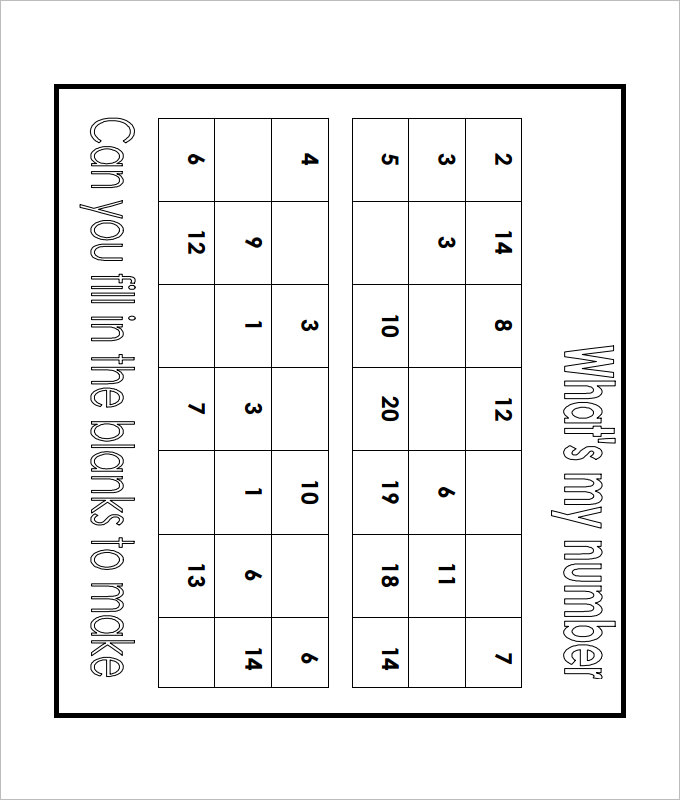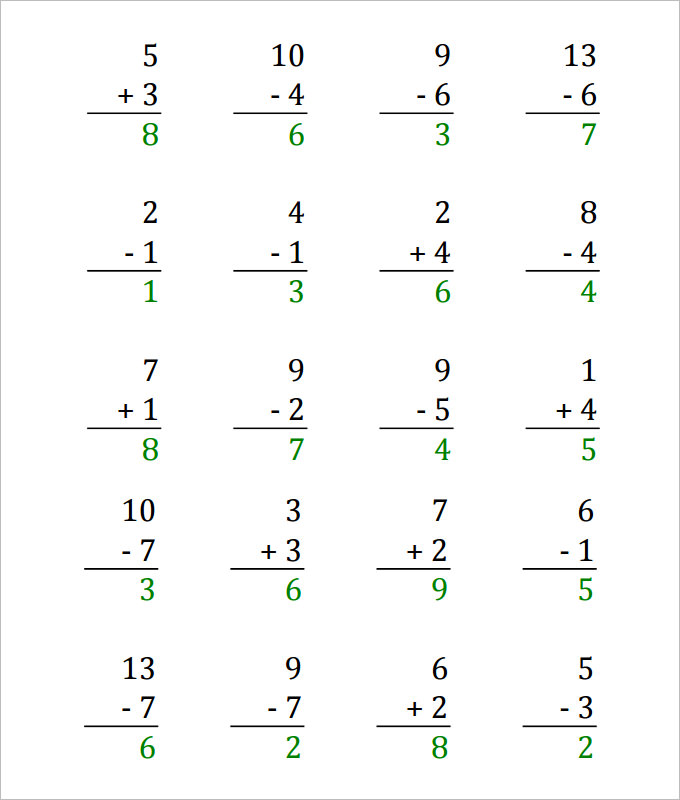## 0 and 10 Addition And Subtraction Worksheet Template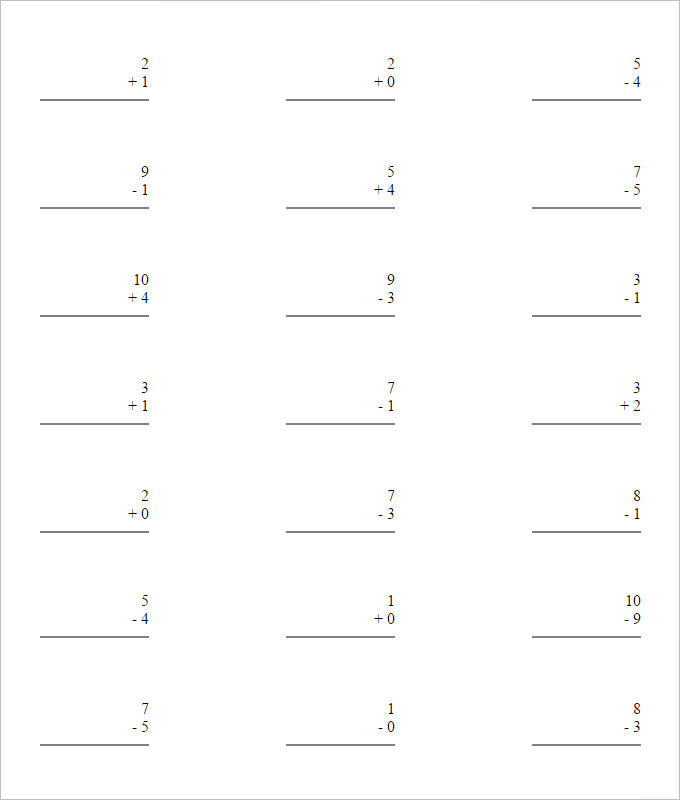## Basic Addition And Subtraction Worksheet Template## 2 Sets Addition And Subtraction Worksheet Template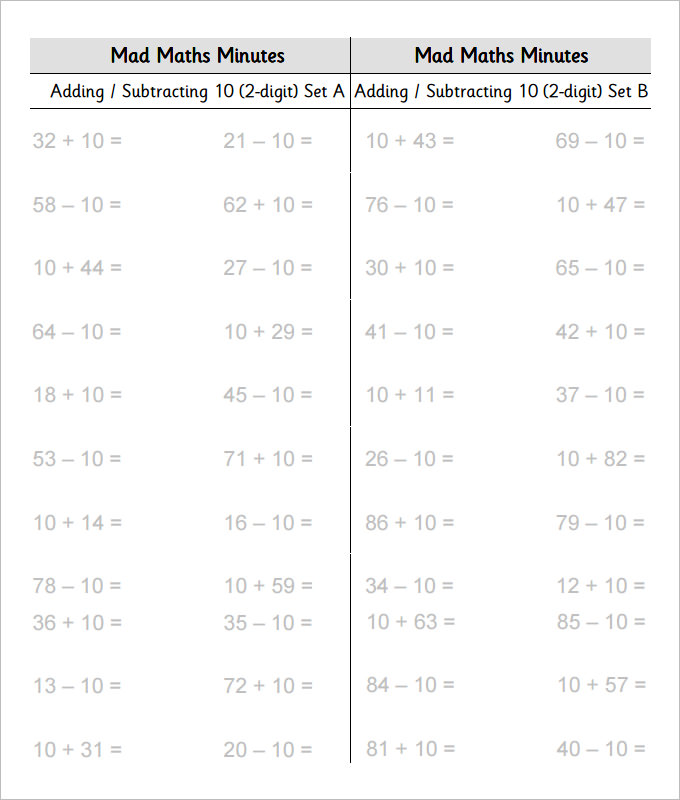Now, creating such worksheets every now and then gets really tough for the maths teachers. But you would be glad to know that today you have sample addition and subtraction worksheets online to relieve much of your burden.

## More on online Addition and Subtraction Worksheets

• There is no need to create a complete worksheet from scratch.
• If you are a primary school teacher, you will find fun maths worksheets online for your little students.
• There are even adding and subtracting fractions worksheets online if you are a High school maths teacher.

### > Why Addition and Subtraction Templates are Important for your Child?

If you, as a parent, have difficulty teaching your kid the rules of subtraction and addition then these templates will help you immensely. Since, they are readily available over the internet, you can have a good collection of them. All you need is to hand one of these worksheets over to your child and see how many problems he can solve correctly. When he completes a template successfully, you can give him a new one with a higher difficulty level so as to assess his progress. This way, your child develops a strong grip over basic mathematics. So, if you want to make your child love maths, you will have to have these templates. They are a great way to let your child practice.

### > Conclusion

There is no dearth of Addition-And-Subtraction-Worksheet templates over the internet. You can search for them and pick the ones that best meet your requirements. With a large variety of options available, you will never run out of choices. What will excite you most about these templates is that most of them are available free of cost. Hence, there is no question of affordability. So, what are you waiting for? Grab as many as you can. Many sites offer free downloads for addition and subtraction worksheets Calculations: Addition & Subtraction Worksheets